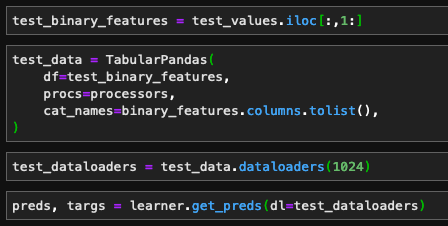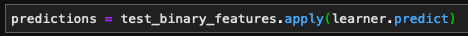# Tabular batch prediction with fastai v2

I have trained a `tabular_learner` on a tabular dataset, and I would now like to invoke the model with a test dataset.

I attempted to do this using `learner.get_preds`:But I ran into a couple of errors:

I then decided to try just predicting row by row, using `pandas.DataFrame.apply`:But I got a `KeyError`:

``````KeyError: "None of [Index([ <column names printed here> ],\n      dtype='object', name=<index column name printed here>)] are in the [columns]"
``````

Then, I decided to just try iterating over the rows in the dataframe:

But this is extremely slow and it seems like it would take most of a day to complete executing.

What is the correct way to get batch predictions for a test dataset with a `TabularLearner` in fastai v2? If anyone can point me in the right direction, I’d really appreciate it!

Hi @ajka

This should work (I used it for `text_classifier_learner` but it should be the same for tabular):

``````test_dl = learner.dls.test_dl(test_binary_features)
predictions = learner.get_preds(dl=test_dl)``````
4 Likes

That worked perfectly. Thanks again, @stefan-ai!

1 Like

Thanks for your reply. However, I have a question. For the new dataset when we do not know the output (label column) of the data, then the predictions for the label part throws None. I think something is wrong here. Because with standard predict method we get the probability+label (for example “yes”/“no”). What do you think?

``````[]
workclass  education  marital-status  ...    fnlwgt  education-num  salary
0        5.0        8.0             3.0  ... -0.837419       0.753672     1.0

[1 rows x 11 columns]

[]
tensor(1)

[]
tensor([0.4342, 0.5658])
``````

Update: probabilities with predict and your suggestion are right but can we get the labels as well?

@henry090: `learn.predict()` and `learn.get_preds()` give different outputs. `learn.predict()` returns predicted class names, predicted class index and predicted probabilities. `learn.get_preds()` on the other hand returns predicted probabilities and true classes. So for a new dataset without labels, it’s correct that the second output returns None.

You can then take the predicted probabilities, get the predicted class index using `argmax` and the corresponding class name by indexing into `dls.vocab` (or `dls.vocab` in NLP).

`fastinference` will do this for you BTW: See here

(for some reason it’s not showing it but you should be able to pass `with_decoded=True` to `get_preds`)

1 Like

@stefan-ai @muellerzr Both answers are very helpful! `dls.vocab` for column names and `with_decoded=True` which will append labels as well! Thanks very much!

Hi @stefan-ai , @muellerzr . When we specify as metric `roc auc binary`, then while predicting a single row I get :

``````  ValueError: Only one class present in y_true. ROC AUC score is not defined in that case.
``````

Is there a way to skip AUC score evaluation while predicting single rows?

@henry090 you should be able to remove it from learn.metrics

1 Like